Hessian matrix

This article describes an analogue for functions of multiple variables of the following term/fact/notion for functions of one variable: second derivative

Definition

Definition in terms of Jacobian matrix and gradient vector

Suppose$f$ is a real-valued function of$n$ variables$x_1,x_2,\dots,x_n$. The Hessian matrix of$f$ is a$n \times n$-matrix-valued function with domain a subset of the domain of$f$, defined as follows: the Hessian matrix at any point in the domain is the Jacobian matrix of the gradient vector of$f$ at the point. In point-free notation, we denote by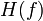$H(f)$ the Hessian matrix function, and we define it as:$H(f) = J(\nabla f)$

Interpretation as second derivative

The Hessian matrix function is the correct notion of second derivative for a real-valued function of$n$ variables. Here's why:

• The correct notion of first derivative for a scalar-valued function of multiple variables is the gradient vector, so the correct notion of first derivative for$f$ is$\nabla f$.
• The gradient vector$\nabla f$ is itself a vector-valued function with$n$-dimensional inputs and$n$-dimensional outputs. The correct notion of derivative for that is the Jacobian matrix, with$n$-dimensional inputs and outputs valued in$n \times n$-matrices.

Thus, the Hessian matrix is the correct notion of second derivative.

Relation with second-order partial derivatives

For further information, refer: Relation between Hessian matrix and second-order partial derivatives

Wherever the Hessian matrix for a function exists, its entries can be described as second-order partial derivatives of the function. Explicitly, for a function$f$ is a real-valued function of$n$ variables$x_1,x_2,\dots,x_n$, the Hessian matrix$H(f)$ is a$n \times n$-matrix-valued function whose$(ij)^{th}$ entry is the second-order partial derivative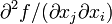$\partial^2f/(\partial x_j\partial x_i)$, which is the same as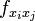$f_{x_ix_j}$. Note that the diagonal entries give second-order pure partial derivatives whereas the off-diagonal entries give second-order mixed partial derivatives.

Some people choose to define the Hessian matrix as the matrix whose entries are the second-order partial derivatives as indicated here. However, that is not quite the correct definition of Hessian matrix because it is possible for all the second-order partial derivatives to exist but for the function to not be twice differentiable at the point. The main disadvantage of defining the Hessian matrix in the more expansive sense (i.e., in terms of second-order partial derivatives) is that all the important results about the Hessian matrix crucially rely on the function being twice differentiable, so we don't actually gain anything by using the more expansive definition.

Continuity assumptions and symmetric matrix

If we assume that all the second-order mixed partial derivatives are continuous at and around a point in the domain, and the Hessian matrix exists, then the Hessian matrix must be a symmetric matrix by Clairaut's theorem on equality of mixed partials. Note that we don't need to assume for this that the second-order pure partials are continuous at or around the point.

In symbols, for a function$f$ of variables$x_1,x_2,\dots,x_n$, we get: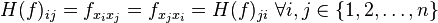$H(f)_{ij} = f_{x_ix_j} = f_{x_jx_i} = H(f)_{ji} \ \forall i,j \in \{ 1,2,\dots,n \}$

Relation with second-order directional derivatives

For further information, refer: Hessian matrix defines bilinear form that outputs second-order directional derivatives

Suppose$f$ is a function of$n$ variables$x_1,x_2,\dots,x_n$, which we think of as a vector variable$\overline{x}$. Suppose$\overline{u},\overline{v}$ are unit vectors in$n$-space. Then, we have the following:$D_{\overline{v}}(D_{\overline{u}}(f)) = \overline{u}^TH(f)\overline{v}$

where$\overline{u},\overline{v}$ are treated as column vectors, so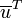$\overline{u}^T$ is$\overline{u}$ as a row vector, and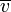$\overline{v}$ is$\overline{v}$ as a column vector. The multiplication on the right side is matrix multiplication. Note that this tells us that the bilinear form corresponding to the Hessian matrix outputs second-order directional derivatives.

Note further that if the second-order mixed partials are continuous, this forces the Hessian matrix to be symmetric, which means that the bilinear form we obtain is symmetric, and hence, we will get:$D_{\overline{v}}(D_{\overline{u}}(f)) = D_{\overline{u}}(D_{\overline{v}}(f))$